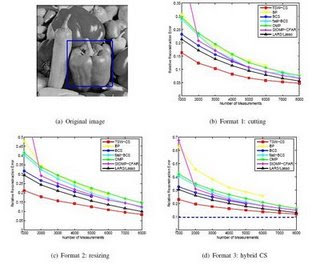Wednesday, January 14, 2009

CS: Bayesian Compressive Sensing, Availability of TSW-CS and BCS-VB codes, Coordinate Gradient Descent Method for l_1-regularized Convex MinimizationBack in October, I mentioned, the following preprint entitled Exploiting structure in wavelet-based bayesian compressed sensing by Lihan He and Lawrence Carin. There is now an implementation of the TSW-CS algorithm available as well as another implementation of the Bayesian Compressive Sensing package at Duke.

• TSW-CS: The TSW-CS implemented by an MCMC approach. The package includes the core TSW-CS code, one example of a 1-dimensional signal and two examples of 2-dimensional images.
Download: tswcs.zip (Last Updated: Dec. 09, 2008)
• VB-BCS: The basic BCS implemented via a variational Bayesian approach. The package includes the core VB-BCS code, one example of a 1-dimensional signal and two examples of 2-dimensional images.

Download: bcs_vb.zip (Last Updated: Dec. 09, 2008)

In applications such as signal processing and statistics, many problems involve finding sparse solutions to under-determined linear systems of equations. These problems can be formulated as a structured nonsmooth optimization problems, i.e., the problem of minimizing l_1-regularized linear least squares problems. In this paper, we propose a block coordinate gradient descent method (abbreviated as CGD) to solve the more general l_1-regularized convex minimization problems, i.e., the problem of minimizing an l_1-regularized convex smooth function. We establish a Q-linear convergence rate for our method when the coordinate block is chosen by a Gauss-Southwell-type rule to ensure sufficient descent. We propose efficient implementations of the CGD method and report numerical results for solving large-scale l_1-regularized linear least squares problems arising in compressed sensing and image deconvolution as well as large-scale l_1-regularized logistic regression problems for feature selection in data classification. Comparison with several state-of-the-art algorithms specifically designed for solving large-scale l_1-regularized linear least squares or logistic regression problems suggests that an efficiently implemented CGD method may outperform these algorithms despite the fact that the CGD method is not specifically designed just to solve these special classes of problems.
Let us note how faster these CGD codes are compared to FPC, GPSR_BB. The authors also made these codes available:
All the links to these codes can be found in the reconstruction section of the big picture.